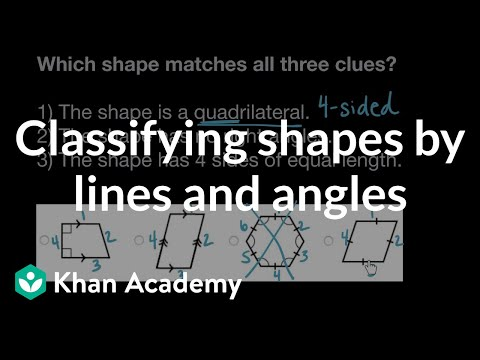Video

# Classifying triangles by angles (Full video)

Description: You say you can't categorize a triangle because you don't know the length of the sides. Guess what? If you know the size of the angles you can! Created by Sal Khan. We've already seen that we can categorize triangles as being equilateral, isoceles, or scalene based on the lengths of the sides of the triangle. If I have at least two of the sides being equal, so let's say that side is the same length as that side right over there, so I'll mark it off as these are the same length, this would be an isosceles triangle. While this one, if we assume this third side is a different length, this would just be isosceles, not equilateral.

### Other videos you might be interested in### Classifying shapes by lines and angles (Full video)